PRIMARY

2 - 6

SECONDARY

7 - 10

SENIOR

11 - 12

# maths tutoring ACT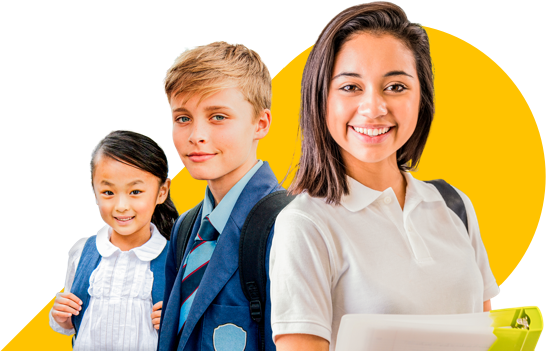## Private Mathematics tutoring for school students in ACT### Expert tutoring

Highly skilled tutors that are friendly, patient and relatable.### Quality content

Quality content designed by education professionals in English, Maths and Chemistry.### Online

Delivered face-to-face online - so you can relax at home while they learn.

#### Meet some of our 210+ expert Mathematics tutors

Our tutors are all qualified teachers, exceptional ATAR achievers or experts in their respective fields.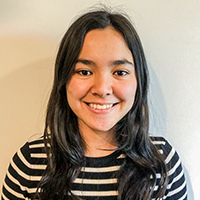#### Angela

ACT University Student

Bachelor of Commerce

Mathematics: Year 7 - Year 10

Angela completed an International Baccalaureate in 2018, with a top ATAR equivalent. She's currently working towards a Bachelor of Commerce and Bachelor of International Relations.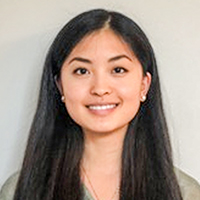#### Emily

VIC University Student

Bachelor of Science

Mathematics: Year 10 - Year 12

Chemistry: Year 10 - Year 12

Originally from the UK, Emily achieved an ATAR of 99 with particular success in extension courses. Emily's expertise are best applied to senior students looking to excel.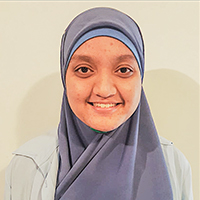#### Iman

NSW University Student

Mathematics: Year 7 - Year 10

Iman completed Advanced Maths in Year 11 and scored top marks for Maths Extension 1 and 2 during her HSC year. She is now studying a Bachelor of Diagnostic Radiology in Sydney.

Related Searches: maths tutoring baulkham hills , maths tutoring beverly hills

## Some of the subjects we cover

Each subject is covered using a learning program that contains all the content you need and is led by your personally matched tutor

Our Year 10 Mathematics Advanced program provides each student with an opportunity to review and build on mathematical areas of study introduced in our Year 9 Mathematics program. Students extend their algebraic techniques to help solve more complex equations and we introduce them to advanced topics including surds, logarithms, polynomials, circle geometry and functions. Each of these topics will be further developed in the advanced senior mathematics courses.

Our Year 10 Mathematics Advanced program provides each student with an opportunity to review and build on mathematical areas of study introduced in our Year 9 Mathematics program. Students extend their algebraic techniques to help solve more complex equations and we introduce them to advanced topics including surds, logarithms, polynomials, circle geometry and functions. Each of these topics will be further developed in the advanced senior mathematics courses.

... with an opportunity to review and build on mathematical areas of study introduced in our Year 9 Mathematics program. Students extend their algebraic techniques to help solve more complex equations and we introduce them ...
More details
Request pricing

Mathematics Year 8

In Year 8, students consolidate their algebraic skills and develop more sophisticated ways to represent and communicate their mathematical understanding. They cover the key curriculum components of financial mathematics, ratios and rates, linear and non-linear relationships, data, circles and cylinders and properties of geometrical figures.

In Year 8, students consolidate their algebraic skills and develop more sophisticated ways to represent and communicate their mathematical understanding. They cover the key curriculum components of financial mathematics, ratios and rates, linear and non-linear relationships, data, circles and cylinders and properties of geometrical figures.

... to represent and communicate their mathematical understanding. They cover the key curriculum components of financial mathematics, ratios and rates, linear and non-linear relationships, data, circles and cylinders and ...
More details
Request pricing

Mathematics Year 9

The Year 9 program covers Number and Algebra, Measurement and Geometry, Statistics and Probability, and introduces the study of Trigonometry. Students use appropriate strategies to solve problems and link mathematical ideas and the relevant terminology and notations to explain their thinking processes. Our Year 9 program places a great deal of importance on consolidating prior mathematical knowledge and laying the strongest foundations for senior Mathematics at every level.

The Year 9 program covers Number and Algebra, Measurement and Geometry, Statistics and Probability, and introduces the study of Trigonometry. Students use appropriate strategies to solve problems and link mathematical ideas and the relevant terminology and notations to explain their thinking processes. Our Year 9 program places a great deal of importance on consolidating prior mathematical knowledge and laying the strongest foundations for senior Mathematics at every level.

... to explain their thinking processes. Our Year 9 program places a great deal of importance on consolidating prior mathematical knowledge and laying the strongest foundations for senior Mathematics at every level.
More details
Request pricing

Mathematics Year 2

Students learn about number theory, such as place value, number sequences and rounding numbers, and simple operations with number such as addition and subtraction. They work with fractions and number sequences to gain a thorough understanding of numbers and patterns.

Students learn about number theory, such as place value, number sequences and rounding numbers, and simple operations with number such as addition and subtraction. They work with fractions and number sequences to gain a thorough understanding of numbers and patterns.

Students learn about number theory, such as place value, number sequences and rounding numbers, and simple operations with number such as addition and subtraction. They work with fractions and number sequences to gain a ...
More details
Request pricing

Mathematics Year 3

Students learn operations with numbers that include addition, subtraction, multiplication and division. They develop greater multiplication skills by learning times tables. They work with measurements of length and area, volume and capacity, mass and time, identify 2D and 3D space and angles and start graphing statistics in simple graphs.

Students learn operations with numbers that include addition, subtraction, multiplication and division. They develop greater multiplication skills by learning times tables. They work with measurements of length and area, volume and capacity, mass and time, identify 2D and 3D space and angles and start graphing statistics in simple graphs. Students are starting to learn the appropriate mathematical terminology to explain their problem-solving strategies.

Students learn operations with numbers that include addition, subtraction, multiplication and division. They develop greater multiplication skills by learning times tables. They work with measurements of length and area, ...
More details
Request pricing

## We asked some of our Mathematics students near ACT what they thought of their tutoring sessions: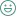Fun
"my session went great i really like my tutor she is really nice to me "
Kulsum, Mathematics student, Yarralumla, ACT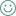Learned A Lot
"i like her a lot just i would like to know where my other tutor went Sarah. but besides that i learnd lots and i give her 5 stars out of five stars "
Thelma, Mathematics student, Yarralumla, ACTFun
"it went well it was fun he is nice and i had a ton of fun "
Kesia, Mathematics student, Yarralumla, ACTFun
"well he so nice and he teaches me stuff that i do not know or understand at school "
Briar, Mathematics student, Yarralumla, ACTLearned A Lot
"We went over fractions on a number line and also we did naplan :DDD "
Dwitika, Mathematics student, Curtin, ACT

## Students from schools across ACT choose Cluey for private tutoring

Burgmann Anglican School
Independent Co-Ed in Gungahlin ACT offering K-12 with a Student to Fulltime Teacher ratio of 12:1
Canberra Grammar School
Independent Co-Ed in Red hill ACT offering K-12 with a Student to Fulltime Teacher ratio of 11:1
Lyneham High School
Government Co-Ed in Lyneham ACT offering 7-10 with a Student to Fulltime Teacher ratio of 14:1
St Francis Xavier College
Catholic Co-Ed in Florey ACT offering 7-12 with a Student to Fulltime Teacher ratio of 13:1
St Mary MacKillop College
Catholic Co-Ed in Isabella plains ACT offering 7-12 with a Student to Fulltime Teacher ratio of 13:1
Telopea Park School
Government Co-Ed in Barton ACT offering K-10 with a Student to Fulltime Teacher ratio of 14:1

## Our report card is in and feedback is positive

As featured in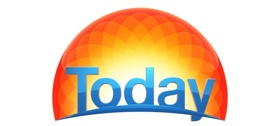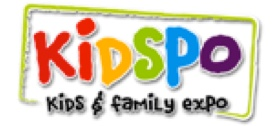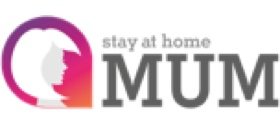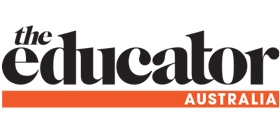## Request Pricing

20% off first two sessionsL8 / 51 Pitt Street Sydney NSW 2000

Phone: 1300 182 000

ABN: 33 620 549 019